# PHP Quiz: All Array Functions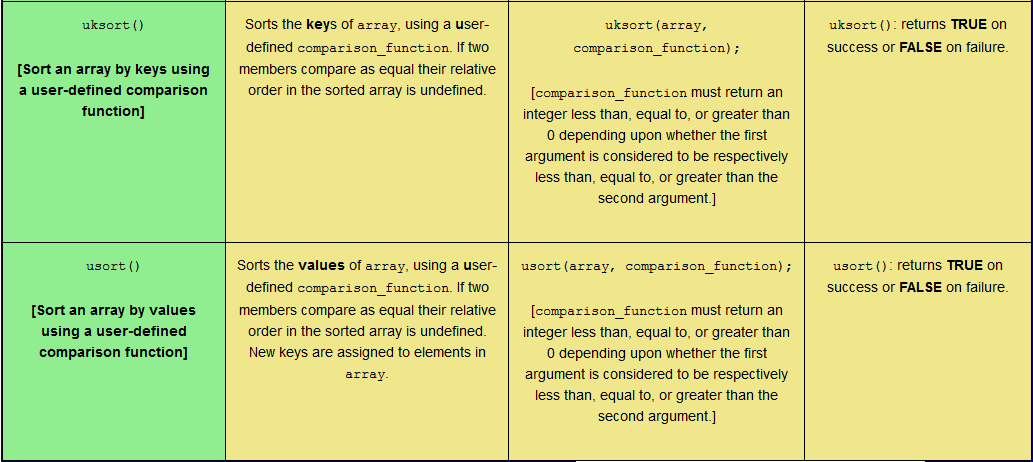This post serves as a reference point for 76 PHP array functions. Users can also click a ‘RANDOMIZE’ button, whereupon all the function descriptions, function syntaxes, and function return values are randomized. The user can then rearrange the table cells correctly (thus completing the quiz) or click an ‘AUTO COMPLETE’ button (following further optional manual randomization) and watch the computer complete the quiz itself! The computer completes the quiz at a speed that the human eye can follow, thus aiding learning.

Tagged with: , , , ,
Posted in PHP Array Quizzes

# PHP Array Function Quiz: `reset()`; `rsort()`; `shuffle()`; `sort()`; `uasort()`; `uksort()`; and `usort()`.

This web page provides a reference table and a subsequent quiz to aid learning seven PHP array functions: `reset()`; `rsort()`; `shuffle()`; `sort()`; `uasort()`; `uksort()`; and `usort()`. The quiz involves matching the function description, function syntax, and function return value to the correct function name. Different table formats are available in order to cater for users on a desktop computer, a laptop, or a mobile phone. Users can also choose to randomize the quiz elements as they see fit before clicking an ‘AUTO COMPLETE’ button and watching the computer correctly complete the quiz!

Tagged with: , , , ,
Posted in PHP Array Quizzes

# PHP Array Function Quiz: `ksort()`; `list()`; `natcasesort()`; `natsort()`; `next()`; `prev()`; and `range()`.

This web page provides a reference table and a subsequent quiz to aid learning seven PHP array functions: `ksort()`; `list()`; `natcasesort()`; `natsort()`; `next()`; `prev()`; and `range()`. The quiz involves matching the function description, function syntax, and function return value to the correct function name. Different table formats are available in order to cater for users on a desktop computer, a laptop, or a mobile phone. Users can also choose to randomize the quiz elements as they see fit before clicking an ‘AUTO COMPLETE’ button and watching the computer correctly complete the quiz!

Tagged with: , , , ,
Posted in PHP Array Quizzes

# PHP Array Function Quiz: `current()`; `each()`; `end()`; `extract()`; `in_array()`; `key()`; and `krsort()`.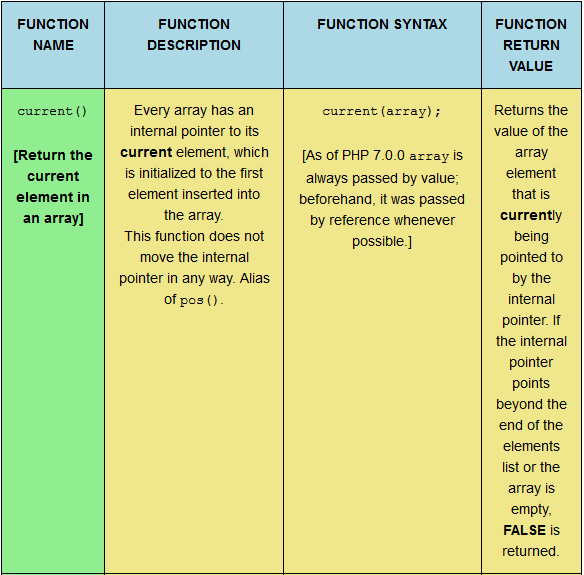This web page provides a reference table and a subsequent quiz to aid learning seven PHP array functions: `current()`; `each`; `end()`; `extract()`; `in_array()`; `key()`; and `krsort()`. The quiz involves matching the function description, function syntax, and function return value to the correct function name. Different table formats are available in order to cater for users on a desktop computer, a laptop, or a mobile phone. Users can also choose to randomize the quiz elements as they see fit before clicking an ‘AUTO COMPLETE’ button and watching the computer correctly complete the quiz!

Tagged with: , , , ,
Posted in PHP Array Quizzes

# PHP Array Function Quiz: `array_walk_recursive()`; `array_walk()`; `arsort()`; `asort()`; `compact()`; and `count()`.This web page provides a reference table and a subsequent quiz to aid learning six PHP array functions: `array_walk_recursive()`; `array_walk()`; `array_arsort()`; `array_asort()`; `array_compact()`; and `array_count()`. The quiz involves matching the function description, function syntax, and function return value to the correct function name. Different table formats are available in order to cater for users on a desktop computer, a laptop, or a mobile phone. Users can also choose to randomize the quiz elements as they see fit before clicking an ‘AUTO COMPLETE’ button and watching the computer correctly complete the quiz!

Tagged with: , , , ,
Posted in PHP Array Quizzes

# PHP Array Function Quiz: `array_udiff()`; `array_uintersect_assoc()`; `array_uintersect_uassoc()`; `array_uintersect()`; `array_unique()`; `array_unshift()`; and `array_values()`.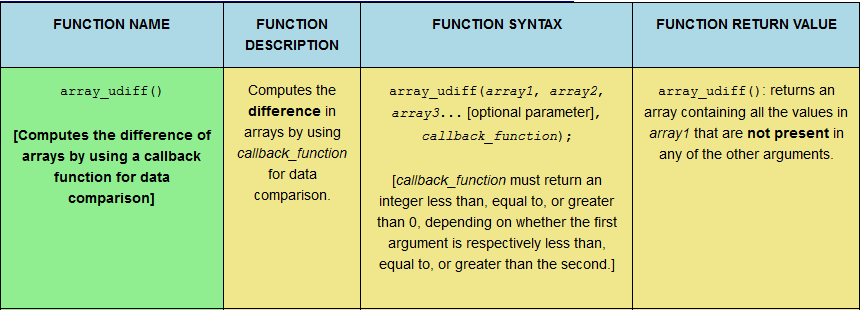This web page provides a reference table and a subsequent quiz to aid learning seven PHP array functions: `array_udiff()`; `array_uintersect_assoc()`; `array_uintersect_uassoc()`; `array_uintersect()`; `array_unique()`; `array_unshift()`; and `array_values()`. The quiz involves matching the function description, function syntax, and function return value to the correct function name. Different table formats are available in order to cater for users on a desktop computer, a laptop, or a mobile phone. Users can also choose to randomize the quiz elements as they see fit before clicking an ‘AUTO COMPLETE’ button and watching the computer correctly complete the quiz!

Tagged with: , , , ,
Posted in PHP Array Quizzes

# PHP Array Function Quiz: `array_search()`; `array_shift()`; `array_slice()`; `array_splice()`; `array_sum()`; `array_udiff_assoc()`; and `array_udiff_uassoc()`.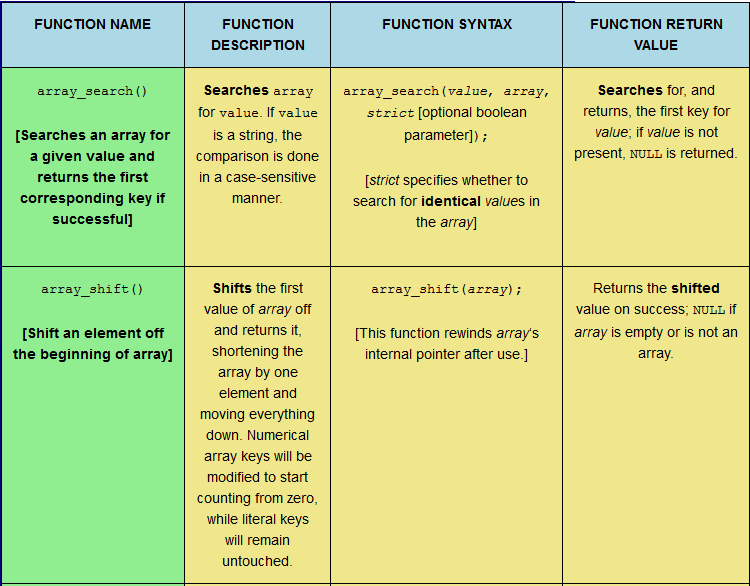This web page provides a reference table and a subsequent quiz to aid learning seven PHP array functions: `array_search()`; `array_shift()`; `array_slice()`; `array_splice()`; `array_sum()`; `array_udiff_assoc()`; and `array_udiff_uassoc()`. The quiz involves matching the function description, function syntax, and function return value to the correct function name. Different table formats are available in order to cater for users on a desktop computer, a laptop, or a mobile phone. Users can also choose to randomize the quiz elements as they see fit before clicking an ‘AUTO COMPLETE’ button and watching the computer correctly complete the quiz!

Tagged with: , , , ,
Posted in PHP Array Quizzes

# PHP Array Function Quiz: `array_product()`; `array_push()`; `array_rand()`; `array_reduce()`; `array_replace_recursive()`; `array_replace()`; and `array_reverse()`.This web page provides a reference table and a subsequent quiz to aid learning seven PHP array functions: `array_product()`; `array_push()`; `array_rand()`; `array_reduce()`; `array_replace_recursive()`; `array_replace()`; and `array_reverse()`. The quiz involves matching the function description, function syntax, and function return value to the correct function name. Different table formats are available in order to cater for users on a desktop computer, a laptop, or a mobile phone. Users can also choose to randomize the quiz elements as they see fit before clicking an ‘AUTO COMPLETE’ button and watching the computer correctly complete the quiz!

Tagged with: , , , ,
Posted in PHP Array Quizzes

# PHP Array Function Quiz: `array_keys()`; `array_map()`; `array_merge_recursive()`; `array_merge()`; `array_multisort()`; `array_pad()`; and `array_pop()`.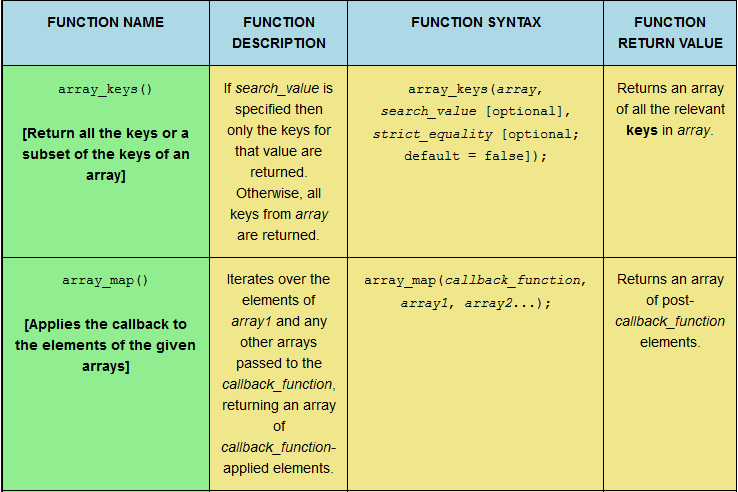This web page provides a reference table and a subsequent quiz to aid learning seven PHP array functions: `array_keys()`; `array_map()`; `array_merge_recursive()`; `array_merge()`; `array_multisort()`; `array_pad()`; and `array_pop()`. The quiz involves matching the function description, function syntax, and function return value to the correct function name. Different table formats are available in order to cater for users on a desktop computer, a laptop, or a mobile phone. Users can also choose to randomize the quiz elements as they see fit before clicking an ‘AUTO COMPLETE’ button and watching the computer correctly complete the quiz!

Tagged with: , , , ,
Posted in PHP Array Quizzes

# PHP Array Function Quiz: `array_flip()`; `array_intersect_assoc()`; `array_intersect_key()`; `array_intersect_uassoc()`; `array_intersect_ukey()`; `array_intersect()`; and `array_key_exists()`.This web page provides a reference table and a subsequent quiz to aid learning seven PHP array functions: `array_flip()`; `array_intersect_assoc()`; `array_intersect_key()`; `array_intersect_uassoc()`; `array_intersect_ukey()`; `array_intersect()`; and `array_key_exists()`. The quiz involves matching the function description, function syntax, and function return value to the correct function name. Different table formats are available in order to cater for users on a desktop computer, a laptop, or a mobile phone. Users can also choose to randomize the quiz elements as they see fit before clicking an ‘AUTO COMPLETE’ button and watching the computer correctly complete the quiz!

Tagged with: , , , ,
Posted in PHP Array Quizzes